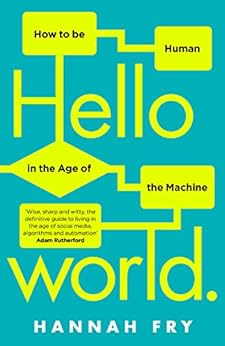#$$\frac{44}{44}$$
$$\frac{4\times4}{4+4}$$
$$\frac{4\times4-4}{4}$$
$$4+4\times(4-4)$$
$$\frac{4}{4}+\sqrt4+\sqrt4$$
$$\frac{4}{4}\times4+\sqrt4$$
$$\frac{44}{4}-4$$
$$4+4+4-4$$
$$4+4+\frac{4}{4}$$
$$\frac{44-4}{4}$$
$$\frac{44}{\sqrt4+\sqrt4}$$
$$\frac{44+4}{4}$$

A special clock for American Independence Day. It only uses the digit 4.
Can you design a special clock for a different day of the year using a different digit?

## A Mathematics Lesson Starter Of The Day

 Tweet Share
• Transum,
•
• At the time of writing this is a brand new Starter of the Day so please let us know whether you like it by leaving a comment or giving it a score using the buttons below. An alternative way of using this starter is to have it on the screen when the pupils are coming into the room then, after everyone has had chance to see it, turn off the projector and ask the class to reproduce it as best they can. Each number of the clock is replaced by four fours. Happy Independence day to all in the USA.

How did you use this starter? Can you suggest how teachers could present or develop this resource? Do you have any comments? It is always useful to receive feedback and helps make this free resource even more useful for Maths teachers anywhere in the world.

If you don't have the time to provide feedback we'd really appreciate it if you could give this page a score! We are constantly improving and adding to these starters so it would be really helpful to know which ones are most useful. Simply click on a button below:

Excellent, I would like to see more like this
Good, achieved the results I required
Satisfactory
Didn't really capture the interest of the students
Not for me! I wouldn't use this type of activity.

Previous Day | This starter is for 4 July | Next Day

There are of course many ways pupils might respond to this challenge but here is an example of a solution

$$1=\left(\frac{9}{9}\right)^9$$

$$2=\left(\frac{9+9}{9}\right)$$

$$3=\sqrt{9}+9-9$$

$$4=\sqrt{9}+\frac{9}{9}$$

$$5=\sqrt{9}!-\frac{9}{9}$$

$$6=\sqrt{9}\times\sqrt{9}-\sqrt{9}$$

$$7=\sqrt{9}!+\frac{9}{9}$$

$$8=9-\frac{9}{9}$$

$$9=9+9-9$$

$$10=9+\frac{9}{9}$$

$$11=99\div9$$

$$12=9+\frac{9}{\sqrt{9}}$$

This type of challenge has been around for a long time. The first known reference is in a book called "The Schoolmasters Assistant: Being a Compendium of Arithmetic, Both Practical and Theoretical". It was written in 1762 by Thomas Dilworth, an English cleric. Here is the wording as it appeared in the book:$$33+\frac33 = 34$$

Your access to the majority of the Transum resources continues to be free but you can help support the continued growth of the website by doing your Amazon shopping using the links on this page. Below is an Amazon search box and some items chosen and recommended by Transum Mathematics to get you started.

## Hello World

You are buying a (driverless) car. One vehicle is programmed to save as many lives as possible in a collision. Another promises to prioritize the lives of its passengers. Which do you choose?

Welcome to the age of the algorithm, the story of a not-too-distant future where machines rule supreme, making important decisions – in healthcare, transport, finance, security, what we watch, where we go even who we send to prison. So how much should we rely on them? What kind of future do we want?

Hannah Fry takes us on a tour of the good, the bad and the downright ugly of the algorithms that surround us. In Hello World she lifts the lid on their inner workings, demonstrates their power, exposes their limitations, and examines whether they really are an improvement on the humans they are replacing. more...Teacher, do your students have access to computers?Do they have iPads or Laptops in Lessons? Whether your students each have a TabletPC, a Surface or a Mac, this activity lends itself to eLearning (Engaged Learning).Here a concise URL for a version of this page without the comments.

Transum.org/go/?Start=July4

Here is the URL which will take them to a related student activity.

Transum.org/go/?to=onedigitHere is the link to the pairs game based on American-English and British-English mathematical words.

Transum.org/go/?to=MathvsMathsHere is a visual aid for teachers to use when teaching alalogue time.

Transum.org/go/?to=clockFor All: